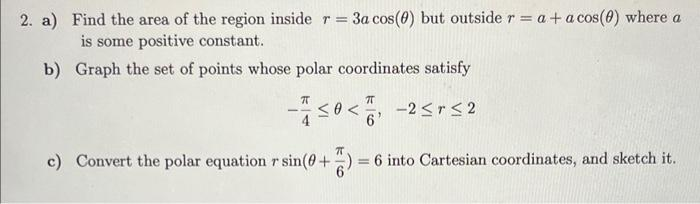# Question 2. a) Find the area of the region inside $$r=3 a \cos (\theta)$$ but outside $$r=a+a \cos (\theta)$$ where $$a$$ is some positive constant. b) Graph the set of points whose polar coordinates satisfy $-\frac{\pi}{4} \leq \theta<\frac{\pi}{6}, \quad-2 \leq r \leq 2$ c) Convert the polar equation $$r \sin \left(\theta+\frac{\pi}{6}\right)=6$$ into Cartesian coordinates, and sketch it.WRK369 The Asker · Chemical EngineeringTranscribed Image Text: 2. a) Find the area of the region inside $$r=3 a \cos (\theta)$$ but outside $$r=a+a \cos (\theta)$$ where $$a$$ is some positive constant. b) Graph the set of points whose polar coordinates satisfy $-\frac{\pi}{4} \leq \theta<\frac{\pi}{6}, \quad-2 \leq r \leq 2$ c) Convert the polar equation $$r \sin \left(\theta+\frac{\pi}{6}\right)=6$$ into Cartesian coordinates, and sketch it.
More
Transcribed Image Text: 2. a) Find the area of the region inside $$r=3 a \cos (\theta)$$ but outside $$r=a+a \cos (\theta)$$ where $$a$$ is some positive constant. b) Graph the set of points whose polar coordinates satisfy $-\frac{\pi}{4} \leq \theta<\frac{\pi}{6}, \quad-2 \leq r \leq 2$ c) Convert the polar equation $$r \sin \left(\theta+\frac{\pi}{6}\right)=6$$ into Cartesian coordinates, and sketch it.&#12304;General guidance&#12305;The answer provided below has been developed in a clear step by step manner.Step1/3a) To find the area of the region inside r = 3a cos(&#952;) but outside r = a + a cos(&#952;), we need to first find the limits of integration for &#952;. These limits are the values of &#952; where the two curves intersect.Setting r = 3a cos(&#952;) equal to r = a + a cos(&#952;), we get:$$\mathrm{{3}{a}{\cos{θ}}={a}+{a}{\cos{θ}}}$$$$\mathrm{{2}{a}{\cos{θ}}={a}}$$$$\mathrm{\theta=\frac{π}{{3}}​,\frac{{{5}π}}{{3}}​}$$So the limits of integration for &#952; are &#960;/3 to 5&#960;/3.The area can then be found using the formula:$$\mathrm{{A}=\frac{{1}}{{2}}{\int_{{\frac{π}{{3}}}}^{{\frac{{{5}π}}{{3}}}}}{\left({3}{a}{\cos{{\left(θ\right)}}}\right)}^{{2}}-{\left({a}+{a}{\cos{{\left(θ\right)}}}\right)}^{{2}}{d}θ}$$Simplifying this expression, we get:$$\mathrm{{A}=\frac{{1}}{{2}}{\int_{{\frac{π}{{3}}}}^{{{5}\frac{π}{{3}}}}}{\left({8}{a}^{{2}}{{\cos}^{{2}}{\left(θ\right)}}-{4}{a}^{{2}}{\cos{{\left(θ\right)}}}-{a}^{{2}}\right)}{d}θ}$$Using the trigonometric identity cos^2(&#952;) = (1 + cos(2&#952;))/2 and simplifying, we get:$$\mathrm{{A}=\frac{{1}}{{4}}{∫_{{\frac{π}{{3}}}}^{{{5}\frac{π}{{3}}}}}{\left({16}{a}^{{2}}{\cos{{\left({2}θ\right)}}}-{8}{a}^{{2}}{\cos{{\left(θ\right)}}}-{3}{a}^{{2}}\right)}{d}θ}$$Integrating this expression, we get:$$\mathrm{{A}=\frac{{5}}{{4}}{a}^{{2}}\pi}$$Explanation:Please refer to solution in this step.Step2/3b. The set of points whose polar coordinates satisfy -&#960;/4 &#8804; &#952; &lt; &#960;/6 and -2 &#8804; r &#8804; 2 can be graphed as a sector of a disk of radius 2, centered at the origin, with central angle &#960;/6 - (-&#960;/4) = 5&#960;/12.To graph this, first draw a disk of radius 2 centered at the origin. Then draw a ray from the origin making an angle of -&#960;/4 with the positive x-axis, and another ray making an angle of &#960;/6 with the positive x-axis. Shade in the region between these two rays, and inside the disk, to obtain the desired sector.<path transform="" transform-origin="119 140" d=" M 222.5 140 A 103.5 103.5 0 1,0 326 243.5 A 103.5 103.5 0 0,0 222.5 140 " fill="#FFFFFF" stroke="rgb(51, 51, 51)" stroke-width="2" stroke-dasharray="0" stroke-linecap="round" stroke-linejoin="round" stroke-miterlimit="10" dominant-baseline="text-before-edge" vector-effect="non-scaling-stroke" data-id="JYGdUTGFSwTosD6e3f47d">yx<path transform="" transform-origin="225.47665405273438 247.01183891296387" d=" M 225.47665405273438 247.01183891296387 L 294.23240756988525 315.76759243011475 " fill="#FFFFFF" stroke="rgb(51, 51, 51)" stroke-width="2" stroke-dasharray="0" stroke-linecap="round" stroke-linejoin="round" stroke-miterlimit="10" dominant-baseline="text-before-edge" vector-effect="non-scaling-stroke" data-id="ndqH5Nw5SDCSEIsO7iynN"><path transform="" transform-origin="225.47665405273438 194.36270141601562" d=" M 225.47665405273438 248.36270141601562 L 314.4766540527344 194.36270141601562 " fill="#FFFFFF" stroke="rgb(51, 51, 51)" stroke-width="2" stroke-dasharray="0" stroke-linecap="round" stroke-linejoin="round" stroke-miterlimit="10" dominant-baseline="text-before-edge" vector-effect="non-scaling-stroke" data-id="-71-YkpnWvGAR21av7g9E">Desired regionExplanation:Please refer to solution in this step.Step3/3To convert the polar equation r sin(&#952; + &#960;/6) = 6 to Cartesian coordinates, we can use the following relationships:$$\mathrm{{x}={r}{\cos{{\left(θ\right)}}}}$$$$\mathrm{{y}={r}{\sin{{\left(θ\right)}}}}$$Substituting r sin(&#952; + &#960;/6) = 6 into the expression for y, we get:$$\mathrm{{y}={\left(\frac{{6}}{{\sin{{\left(θ+\frac{π}{{6}}\right)}}}}\right)}{\sin{{\left(θ\right)}}}}$$Using the trigonometric identity sin(&#952; + ... See the full answer